"Blackbody radiation" or "cavity radiation" refers to an object or system which absorbs all radiation incident upon it and re-radiates energy which is characteristic of this radiating system only, not dependent upon the type of radiation which is incident upon it. The radiated energy can be considered to be produced by standing wave or resonant modes of the cavity which is radiating.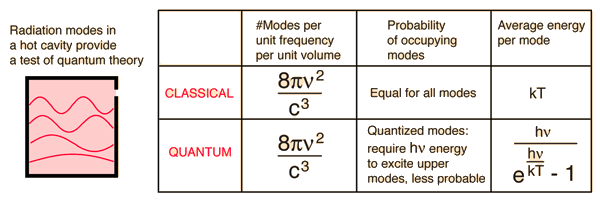The amount of radiation emitted in a given frequency range should be proportional to the number of modes in that range. The best of classical physics suggested that all modes had an equal chance of being produced, and that the number of modes went up proportional to the square of the frequency. But the predicted continual increase in radiated energy with frequency (dubbed the "ultraviolet catastrophe") did not happen. Nature knew better.### Why more modes at higher frequency?

Index

Great experiments of physics

 HyperPhysics***** Quantum Physics R Nave
Go Back

# Cavity Modes

A mode for an electromagnetic wave in a cavity must satisfy the condition of zero electric field at the wall. If the mode is of shorter wavelength, there are more ways you can fit it into the cavity to meet that condition. Careful analysis by Rayleigh and Jeans showed that the number of modes was proportional to the frequency squared.Evaluation of the number of modes
Index

 HyperPhysics***** Quantum Physics R Nave
Go Back

From the assumption that the electromagnetic modes in a cavity were quantized in energy with the quantum energy equal to Planck's constant times the frequency, Planck derived a radiation formula. The average energy per "mode" or "quantum" is the energy of the quantum times the probability that it will be occupied (the Einstein-Bose distribution function):This average energy times the density of such states, expressed in terms of either frequency or wavelength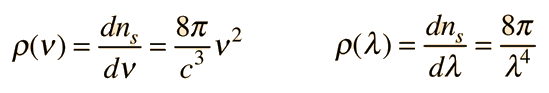gives the energy density, the Planck radiation formula.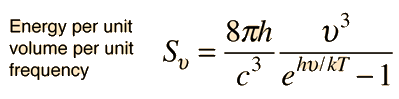### Example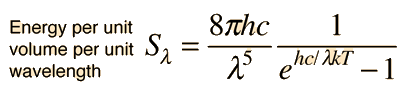### Example

The Planck radiation formula is an example of the distribution of energy according to Bose-Einstein statistics. The above expressions are obtained by multiplying the density of states in terms of frequency or wavelength times the photon energy times the Bose-Einstein distribution function with normalization constant A=1.

To find the radiated power per unit area from a surface at this temperature, multiply the energy density by c/4. The density above is for thermal equilibrium, so setting inward=outward gives a factor of 1/2 for the radiated power outward. Then one must average over all angles, which gives another factor of 1/2 for the angular dependence which is the square of the cosine.

 Major applications of the Planck formula
Index

 HyperPhysics***** Quantum Physics R Nave
Go Back

# Rayleigh-Jeans vs Planck

Comparison of the classical Rayleigh-Jeans Law and the quantum Planck radiation formula. Experiment confirms the Planck relationship.### Comments on the development of the Rayleigh-Jeans Law

 Why does the Planck curve drop below the Rayleigh-Jeans? Frequency plot Show that the two agree for long wavelengths
Index

 HyperPhysics***** Quantum Physics R Nave
Go Back

# Blackbody Intensity as a Function of Frequency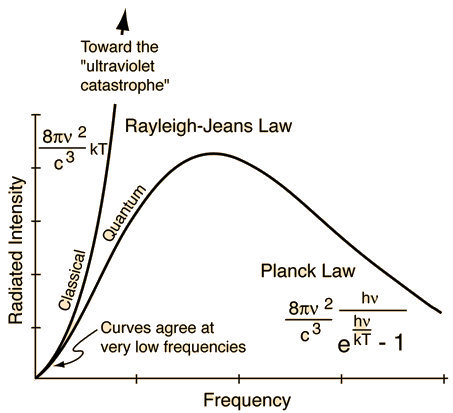### Wavelength plot

Index

 HyperPhysics***** Quantum Physics R Nave
Go Back

# Comments on the Development of the Rayleigh-Jeans Law

The Rayleigh-Jeans Law was an important step in our understanding of the equilibrium radiation from a hot object, even though it turned out not to be an accurate description of nature. The careful work in developing the Rayleigh-Jeans law laid the foundation for the quantum understanding expressed in the Planck radiation formula. In outline form, here are the steps which led to the Rayleigh-Jeans law.
 Equilibrium standing wave electromagnetic radiation in a cubical cavity of dimension L must meet the condition:Show The number of modes in the cavity is: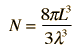Show The number of modes per unit wavelength is: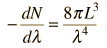Show The energy per unit volume per unit wavelength is: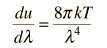Show The average radiated energy per unit wavelength is: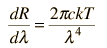Show Which when expressed in terms of frequency is:Show